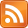The mean of a set of numbers $x_1, x_2 \ldots x_n$ is the unique value $\overline{x}$ which minimizes $\sum (\overline{x} - x_i)^2$, the sum of squared distances between it and the numbers. Why? The mean seems very natural — for example, it's related to intuitive ideas about fairness — but the sum of squares less so. For instance, what's so special about squaring? Why not the sum of fourth powers $\sum (\overline{x} - x_i)^4$ ? Or the sum of unsigned distances $\sum |\overline{x} - x_i|$ ?